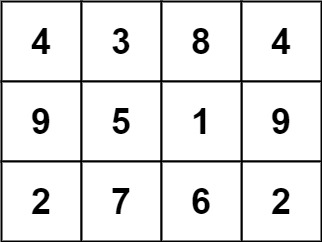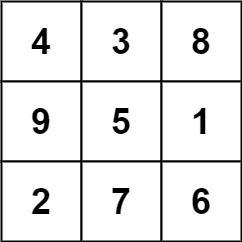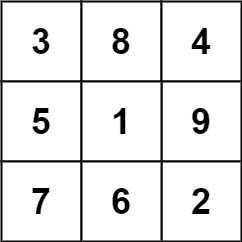# GeetCode Hub

A `3 x 3` magic square is a `3 x 3` grid filled with distinct numbers from `1` to `9` such that each row, column, and both diagonals all have the same sum.

Given a `row x col` `grid` of integers, how many `3 x 3` "magic square" subgrids are there?  (Each subgrid is contiguous).

Example 1:```Input: grid = [[4,3,8,4],[9,5,1,9],[2,7,6,2]]
Output: 1
Explanation:
The following subgrid is a 3 x 3 magic square:while this one is not:In total, there is only one magic square inside the given grid.
```

Example 2:

```Input: grid = []
Output: 0
```

Example 3:

```Input: grid = [[4,4],[3,3]]
Output: 0
```

Example 4:

```Input: grid = [[4,7,8],[9,5,1],[2,3,6]]
Output: 0
```

Constraints:

• `row == grid.length`
• `col == grid[i].length`
• `1 <= row, col <= 10`
• `0 <= grid[i][j] <= 15`

class Solution { public int numMagicSquaresInside(int[][] grid) { } }